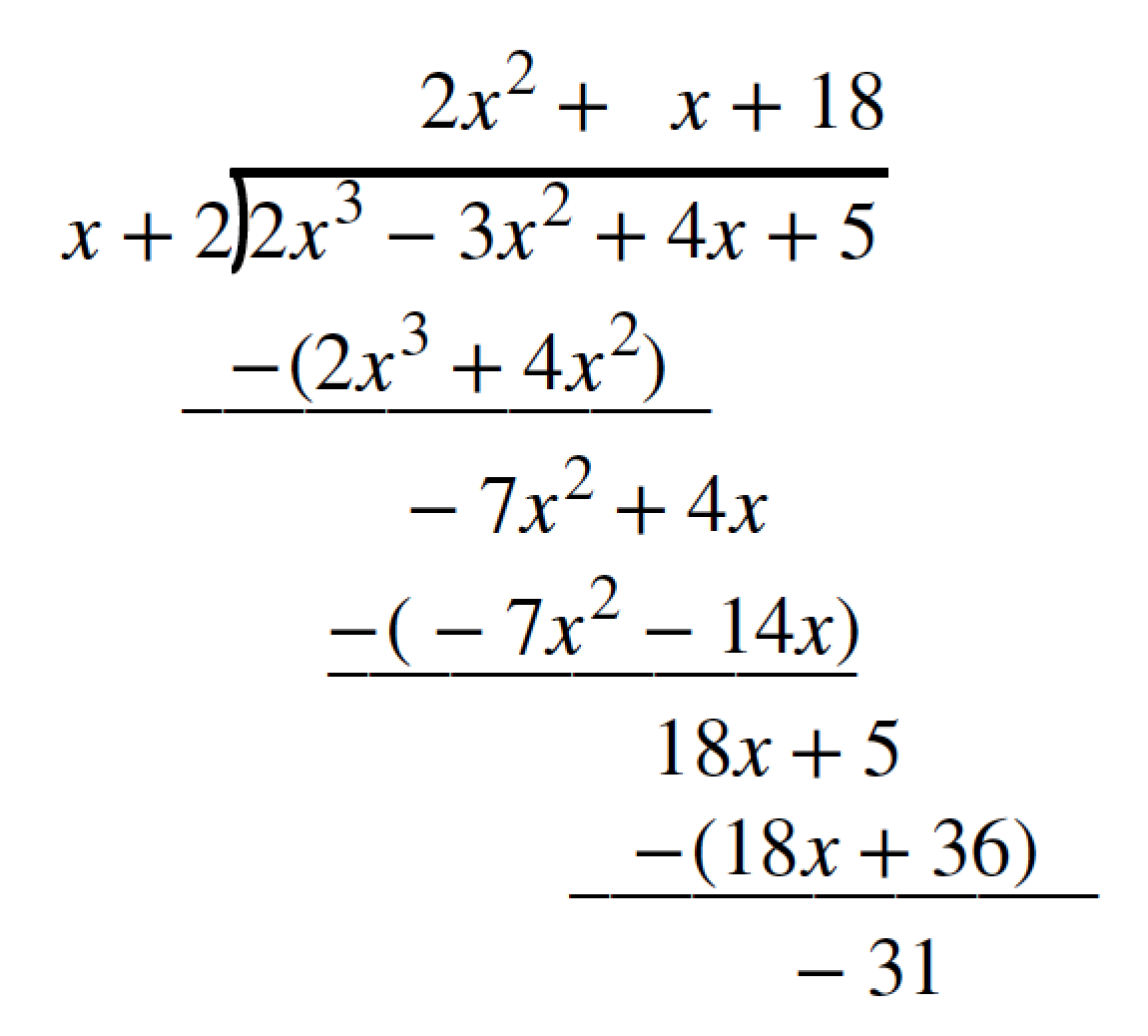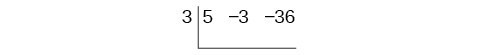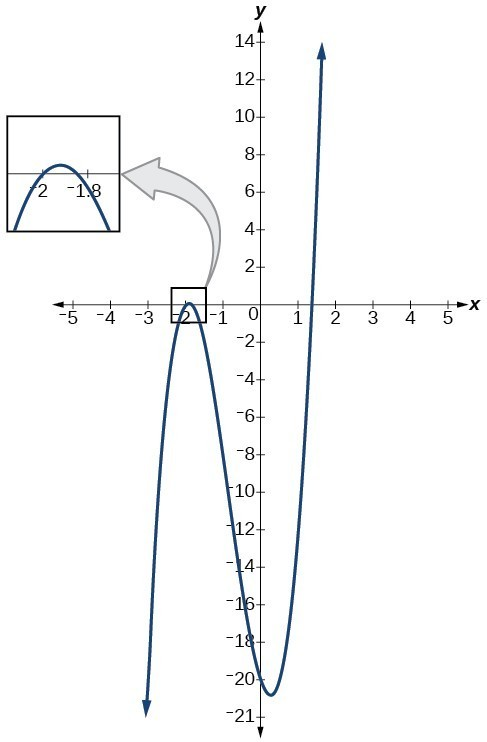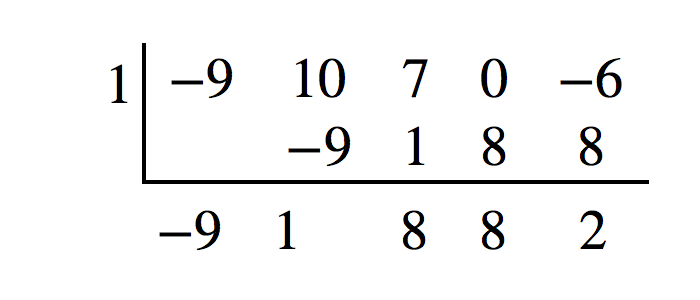## Use synthetic division to divide polynomials

As we’ve seen, long division of polynomials can involve many steps and be quite cumbersome. Synthetic division is a shorthand method of dividing polynomials for the special case of dividing by a linear factor whose leading coefficient is 1.

To illustrate the process, recall the example at the beginning of the section.

Divide $2{x}^{3}-3{x}^{2}+4x+5$ by $x+2$ using the long division algorithm.

The final form of the process looked like this:There is a lot of repetition in the table. If we don’t write the variables but, instead, line up their coefficients in columns under the division sign and also eliminate the partial products, we already have a simpler version of the entire problem.Synthetic division carries this simplification even a few more steps. Collapse the table by moving each of the rows up to fill any vacant spots. Also, instead of dividing by 2, as we would in division of whole numbers, then multiplying and subtracting the middle product, we change the sign of the “divisor” to –2, multiply and add. The process starts by bringing down the leading coefficient.We then multiply it by the “divisor” and add, repeating this process column by column, until there are no entries left. The bottom row represents the coefficients of the quotient; the last entry of the bottom row is the remainder. In this case, the quotient is $2x{^2} -7x+18$ and the remainder is –31. The process will be made more clear in Example 3.

### A General Note: Synthetic Division

Synthetic division is a shortcut that can be used when the divisor is a binomial in the form x – k. In synthetic division, only the coefficients are used in the division process.

### How To: Given two polynomials, use synthetic division to divide.

1. Write k for the divisor.
2. Write the coefficients of the dividend.
3. Bring the lead coefficient down.
4. Multiply the lead coefficient by k. Write the product in the next column.
5. Add the terms of the second column.
6. Multiply the result by k. Write the product in the next column.
7. Repeat steps 5 and 6 for the remaining columns.
8. Use the bottom numbers to write the quotient. The number in the last column is the remainder and has degree 0, the next number from the right has degree 1, the next number from the right has degree 2, and so on.

### Example 3: Using Synthetic Division to Divide a Second-Degree Polynomial

Use synthetic division to divide $5{x}^{2}-3x - 36$ by $x - 3.$

### Solution

Begin by setting up the synthetic division. Write k and the coefficients.Bring down the lead coefficient. Multiply the lead coefficient by k.Continue by adding the numbers in the second column. Multiply the resulting number by k. Write the result in the next column. Then add the numbers in the third column.The result is $5x+12.$ The remainder is 0. So $x - 3$ is a factor of the original polynomial.

### Analysis of the Solution

Just as with long division, we can check our work by multiplying the quotient by the divisor and adding the remainder.

$\left(x - 3\right)\left(5x+12\right)+0=5{x}^{2}-3x - 36$

### Example 4: Using Synthetic Division to Divide a Third-Degree Polynomial

Use synthetic division to divide $4{x}^{3}+10{x}^{2}-6x - 20$ by $x+2.$

### Solution

The binomial divisor is $x+2$ so $k=-2.$ Add each column, multiply the result by –2, and repeat until the last column is reached.The result is $4{x}^{2}+2x - 10.$ The remainder is 0. Thus, $x+2$ is a factor of $4{x}^{3}+10{x}^{2}-6x - 20.$

### Analysis of the Solution

The graph of the polynomial function $f\left(x\right)=4{x}^{3}+10{x}^{2}-6x - 20$ in Figure 2 shows a zero at $x=k=-2.$ This confirms that $x+2$ is a factor of $4{x}^{3}+10{x}^{2}-6x - 20.$Figure 2

### Example 5: Using Synthetic Division to Divide a Fourth-Degree Polynomial

Use synthetic division to divide $-9{x}^{4}+10{x}^{3}+7{x}^{2}-6$ by $x - 1.$

### Solution

Notice there is no x-term. We will use a zero as the coefficient for that term.The result is $-9{x}^{3}+{x}^{2}+8x+8+\frac{2}{x - 1}.$

### Try It 2

Use synthetic division to divide $3{x}^{4}+18{x}^{3}-3x+40$ by $x+7.$

Solution